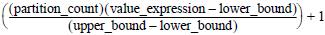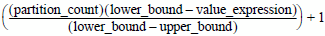# 16.20 - Rules - Teradata Database

## Teradata Vantage™ - SQL Functions, Expressions, and Predicates

prodname
vrm_release
16.20
created_date
March 2019
category
Programming Reference
featnum
B035-1145-162K
The following rules apply to WIDTH_BUCKET:
• If any argument is null, then the result is also null.
• If partition_count <=0 or if partition_count > 2147483646, an error is returned to the requestor.
• If lower_bound = upper_bound, an error is returned to the requestor.
• If lower_bound < upper_bound, then the rules in the following table apply.
IF … THEN the result is …
value_expression < lower_bound 0.
value_expression >= upper_bound partition_count +1.

If the result cannot be represented by the data type specified for the result, then an error is returned.

anything else the greatest exact numeric value with scale 0 that is less than or equal to the following expression.• If lower_bound > upper_bound, then the rules in the following table apply.
IF … THEN the result is …
value_expression > lower_bound 0.
value_expression <= upper_bound partition_count +1.

If the result cannot be represented by the data type specified for the result, then an error is returned.

anything else the least exact numeric value with scale 0 that is less than or equal to the following expression.